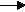gcsescience.com                                       8                                       gcsescience.com

Moles

Calculating the Mass of a Product from a Reaction.
Basic Method.

For a chemical reaction, elements or compounds on
the left side of the equation are called reactants,
those on the right are called products.

reactantsproducts.

Example 1.

What mass of magnesium oxide will be formed
when 10 g  of magnesium is burned in air?

1) Find how many moles of magnesium are present
in 10 g  of magnesium.

The RAM of magnesium is 24.

moles = mass ÷ RFM

moles = 10 ÷ 24
= 0·417 moles of magnesium.

2) Write the equation for
the reaction between magnesium and oxygen
(burning in air means reacting with oxygen).

magnesium + oxygenmagnesium oxide.
2Mg(s)   +   O2(g)2MgO(s)

If you don't know why the formula
for magnesium oxide is MgO, see valency.

Look at the big numbers in front of the elements or compounds.
The big numbers tell you
that 2 magnesiums will give 2 magnesium oxides.
This means that 2 moles of Mg give 2 moles of MgO,
or one mole of Mg gives one mole of MgO.

The big numbers in the equation tell you that
the proportion of Mg to MgO is one to one.

Since the proportion of Mg to MgO is 1 to 1,
then 0·417 moles of magnesium
will give 0·417 moles of magnesium oxide.

3) Find the mass of 0·417 moles of magnesium oxide.

The RFM of magnesium oxide is 40.

mass = moles x RFM

mass = 0·417 x 40
= 16·67 g  of magnesium oxide.

10 g  of magnesium burnt in air
will give 16·67 g  of magnesium oxide (phew!).

The next page will show an advanced method (a shortcut)